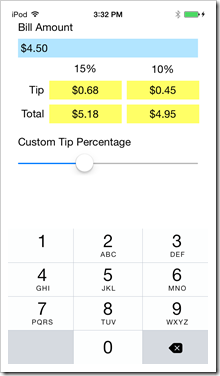I just finished writing my first Swift app for iOS. It’s the very common tutorial app “Tip Calculator” which accepts a bill amount and then shows a tip and total based on the selected tip percentage.

The tutorial is from the book iOS for Programmers: An App-Driven Approach with Swift.

I am not sure if the writers ever built and ran the app, or just wrote the code assuming that it would work. Perhaps the newer xCode 6 beta version supports it, but the non-beta xCode 6 that I’m using doesn’t let me do math with NSDecimalNumber objects without calling appropriate math functions. For instance, a = b + c will not work if a, b, and c are all NSDecimalNumber objects. Instead, the code needs to be a = b.decimalNumberByAdding( c ). How did they get their code to work? When I tried what they had written, the compiler just gave me an error.

I would certainly not give the book 5 stars because there was code on one page that called a function that was not described until two pages later. I like to type in the code as I read and I also like to compile my code often to catch typos. The code won’t compile if the function from two pages later isn’t there yet. But the text seems well written and there were no other problems. So maybe it’s a 4-out-of-5-star book.My Tip Calculator

As for the tip calculator tutorial, I made a few changes. Their user interface places the slider just below the Bill Amount (blue) field and the prompt “Custom Tip Percentage” included the selected percentage. It seemed redundant to show the custom percentage in two places so I got rid of the duplicate number. I then noticed that the custom percentage column was hidden by my finger as I moved the slider, so I move the slider to below the tip and total numbers.

My next modification was to divide the input number by 100 so that the user doesn’t need to press the decimal point. All ATM’s, and many other types of devices that accept dollar amounts, don’t require a decimal point. They just let you enter a number like “120” to represent “\$1.20”. Since this is common, I copied the technique.

My final modification was to how the slider control affects the display. The code in the book would take the number from the slider, divide by 100, and then display it as a percentage. But the calculations of the tip amount used the slider value directly. This means that the custom tip column heading might say “18%” but the tip amount might be 18.2%, not at all the same value. I have to find example code on the internet where NSDecimalNumber values are rounded. There is a lot of code-bloat when doing this because other number handling settings must get set at the same time as the precision and rounding settings. Overall, BSDecimalNumber comes with a lot of baggage; math operators are not overloaded, requiring function calls to do simple math, and formatting takes more effort than I wanted. Plus, the documentation sort of sucks without having even a single line of example code in the reference manuals.